Скачать презентацию Lecture 08 State Feedback Controller Design 8 1

879af3b91c797ecb6e89596ea22b6ee5.ppt

• Количество слайдов: 45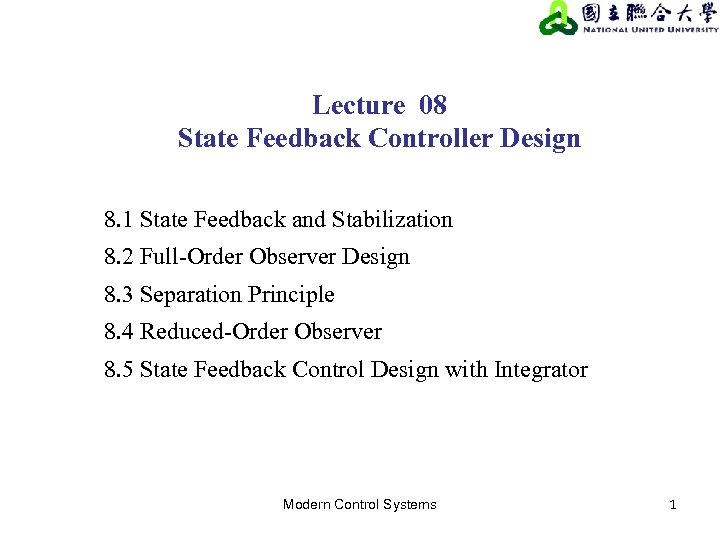Lecture 08 State Feedback Controller Design 8. 1 State Feedback and Stabilization 8. 2 Full-Order Observer Design 8. 3 Separation Principle 8. 4 Reduced-Order Observer 8. 5 State Feedback Control Design with Integrator Modern Control Systems 1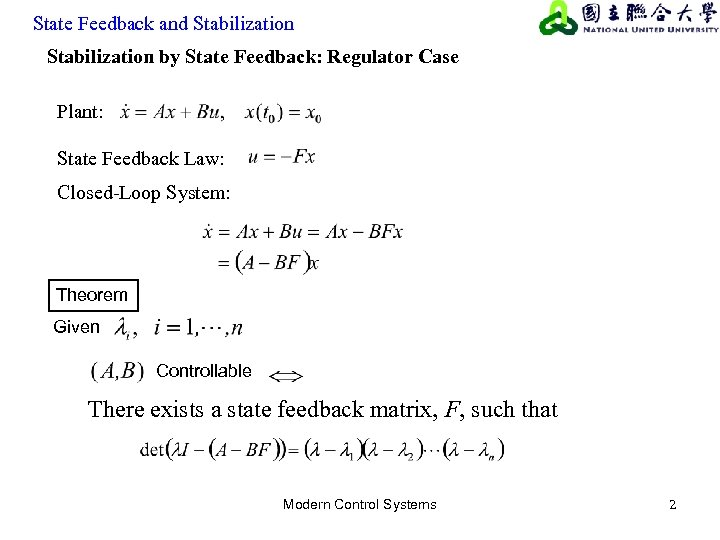State Feedback and Stabilization by State Feedback: Regulator Case Plant: State Feedback Law: Closed-Loop System: Theorem Given Controllable There exists a state feedback matrix, F, such that Modern Control Systems 2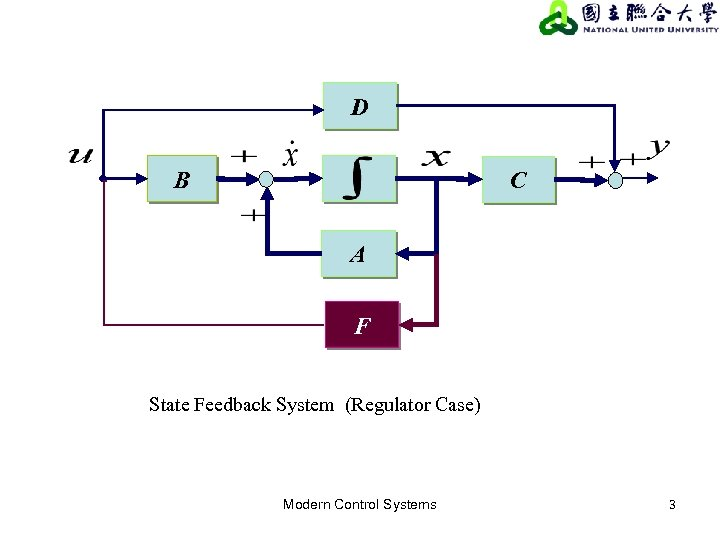D B C A F State Feedback System (Regulator Case) Modern Control Systems 3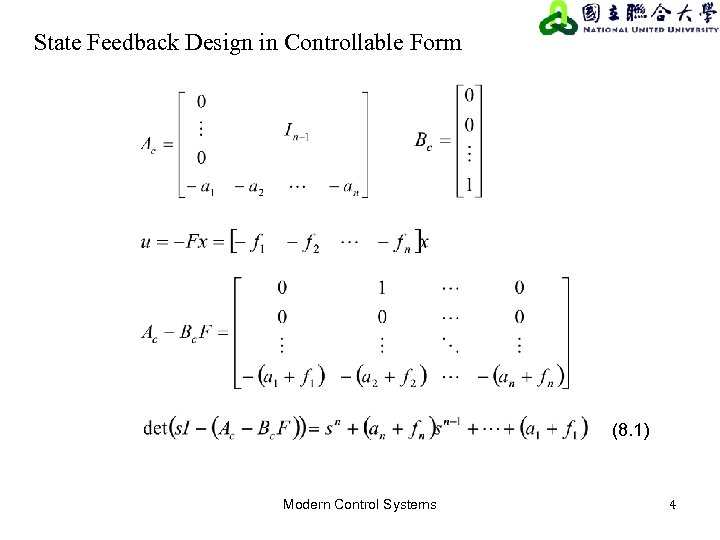State Feedback Design in Controllable Form (8. 1) Modern Control Systems 4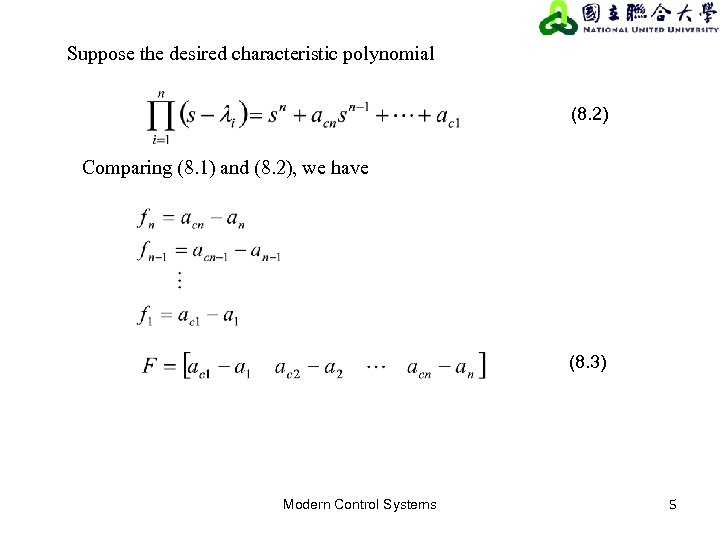Suppose the desired characteristic polynomial (8. 2) Comparing (8. 1) and (8. 2), we have (8. 3) Modern Control Systems 5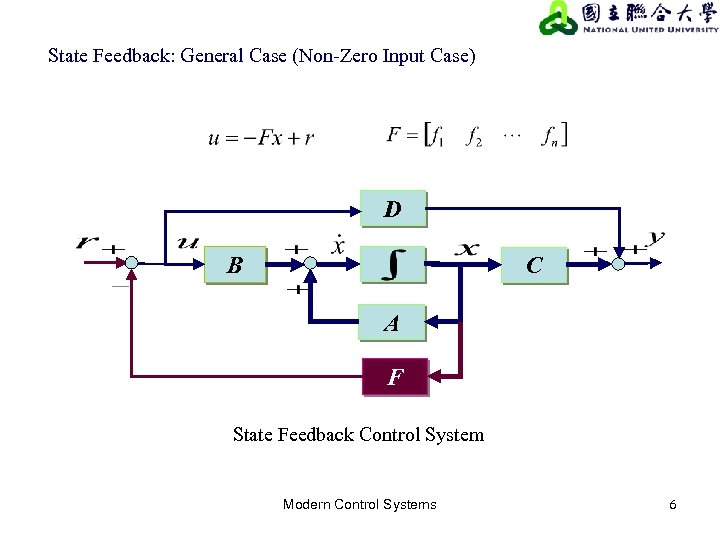State Feedback: General Case (Non-Zero Input Case) D B C A F State Feedback Control System Modern Control Systems 6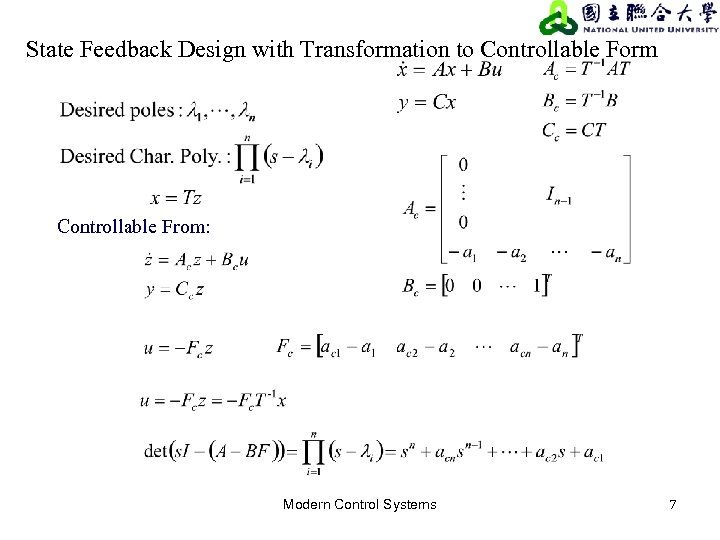State Feedback Design with Transformation to Controllable Form Controllable From: Modern Control Systems 7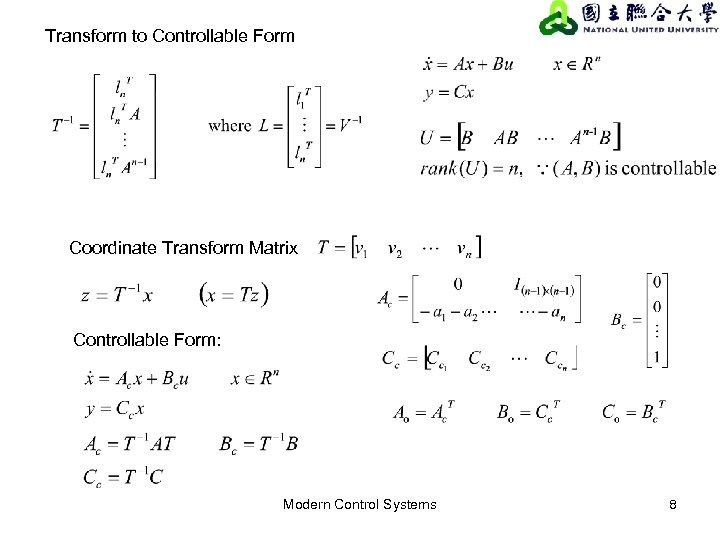Transform to Controllable Form Coordinate Transform Matrix Controllable Form: Modern Control Systems 8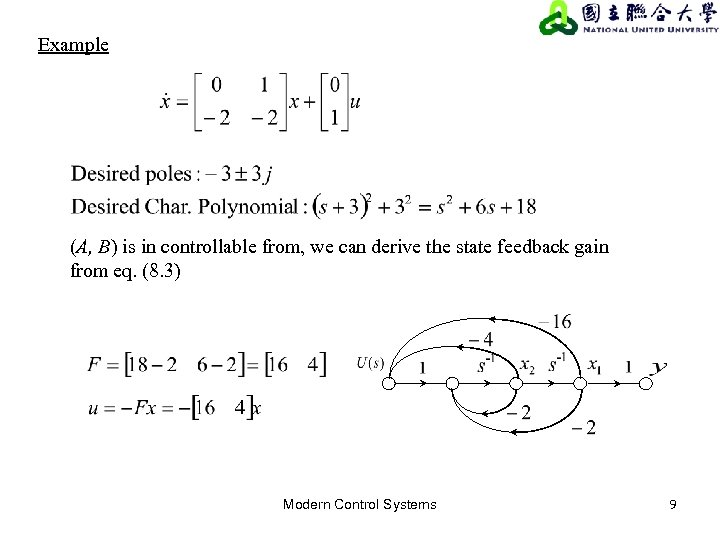Example (A, B) is in controllable from, we can derive the state feedback gain from eq. (8. 3) Modern Control Systems 9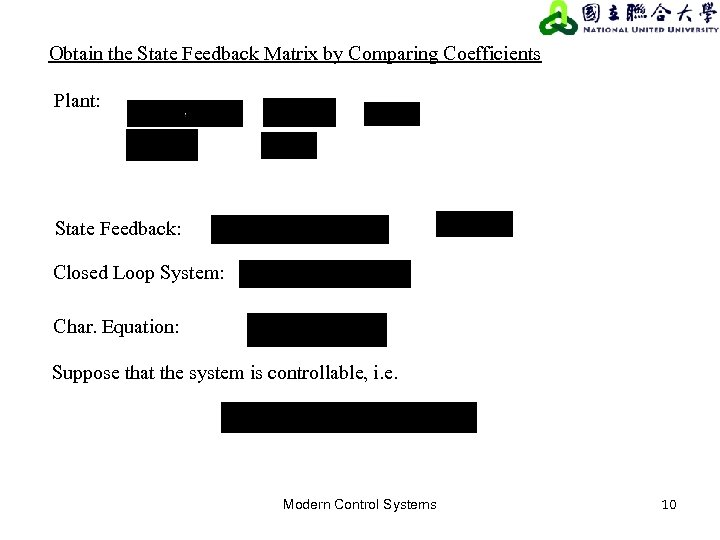Obtain the State Feedback Matrix by Comparing Coefficients Plant: State Feedback: Closed Loop System: Char. Equation: Suppose that the system is controllable, i. e. Modern Control Systems 10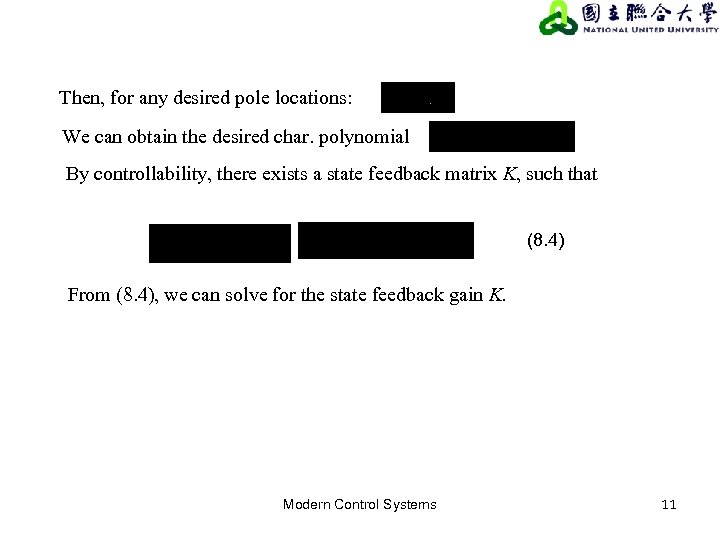Then, for any desired pole locations: We can obtain the desired char. polynomial By controllability, there exists a state feedback matrix K, such that (8. 4) From (8. 4), we can solve for the state feedback gain K. Modern Control Systems 11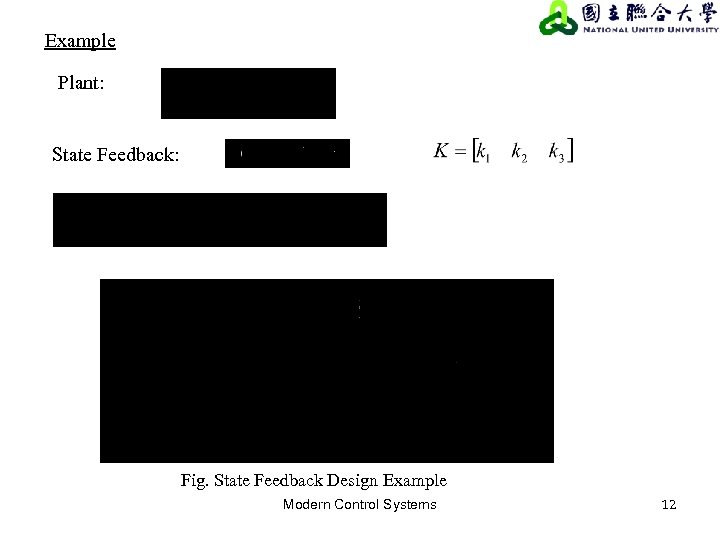Example Plant: State Feedback: Fig. State Feedback Design Example Modern Control Systems 12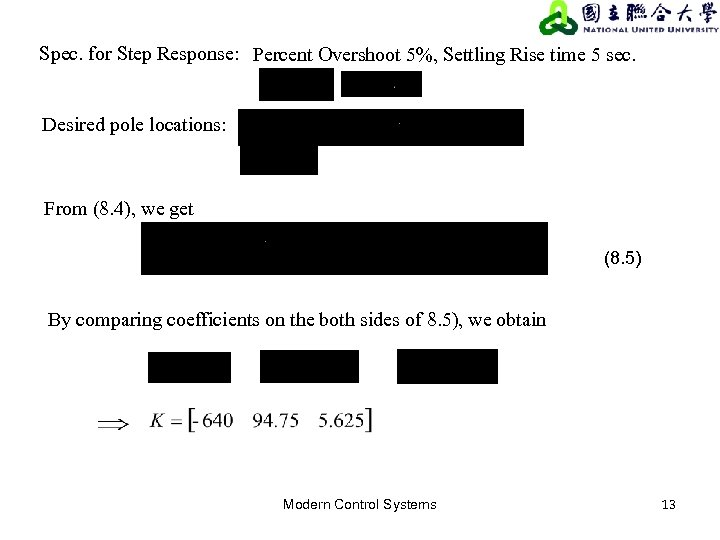Spec. for Step Response: Percent Overshoot 5%, Settling Rise time 5 sec. Desired pole locations: From (8. 4), we get (8. 5) By comparing coefficients on the both sides of 8. 5), we obtain Modern Control Systems 13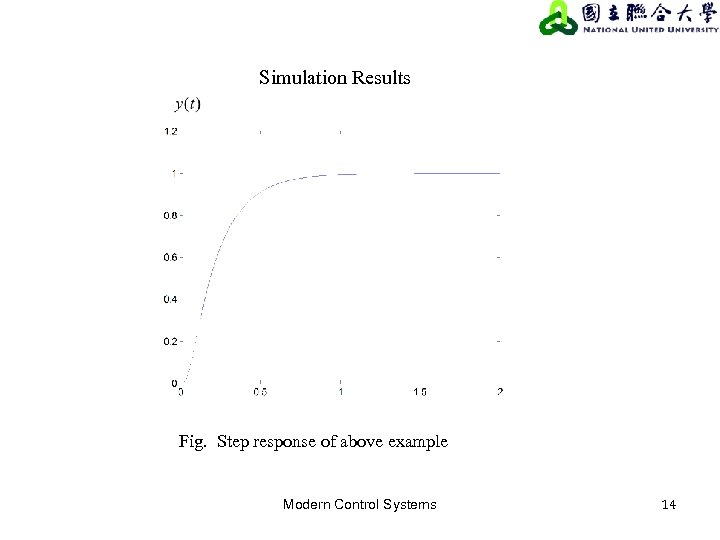Simulation Results Fig. Step response of above example Modern Control Systems 14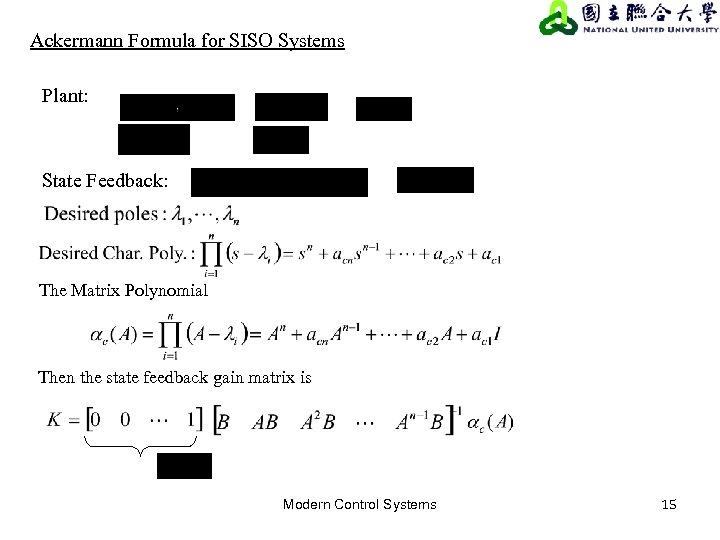Ackermann Formula for SISO Systems Plant: State Feedback: The Matrix Polynomial Then the state feedback gain matrix is Modern Control Systems 15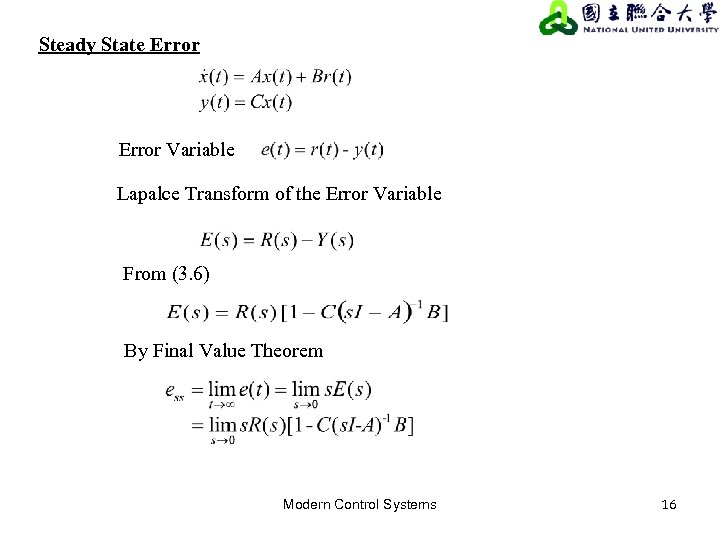Steady State Error Variable Lapalce Transform of the Error Variable From (3. 6) By Final Value Theorem Modern Control Systems 16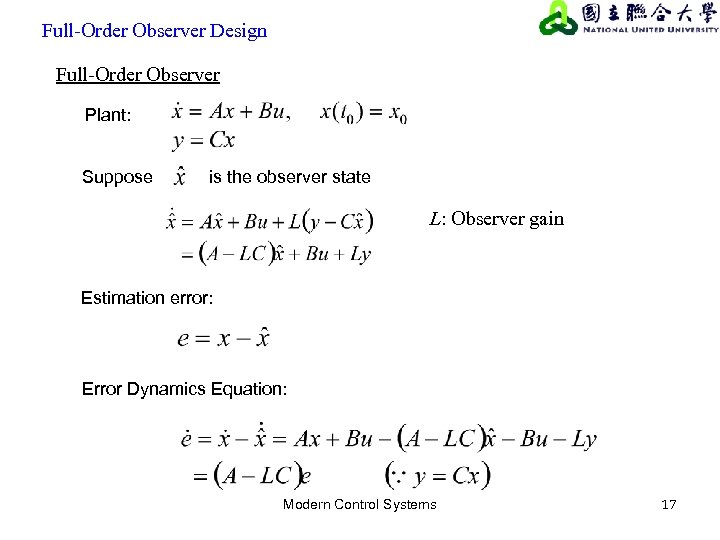Full-Order Observer Design Full-Order Observer Plant: Suppose is the observer state L: Observer gain Estimation error: Error Dynamics Equation: Modern Control Systems 17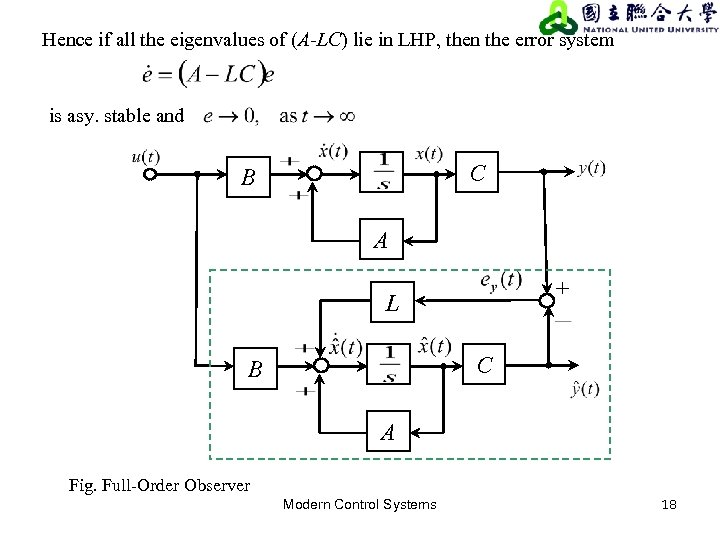Hence if all the eigenvalues of (A-LC) lie in LHP, then the error system is asy. stable and C B A + L C B A Fig. Full-Order Observer Modern Control Systems 18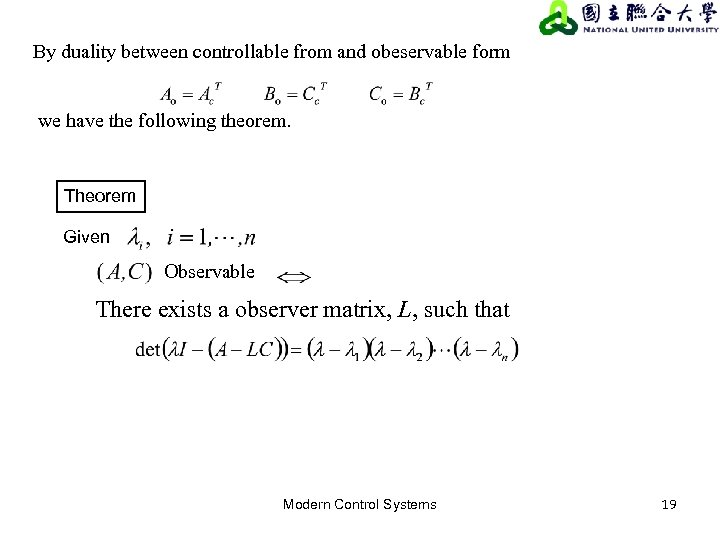By duality between controllable from and obeservable form we have the following theorem. Theorem Given Observable There exists a observer matrix, L, such that Modern Control Systems 19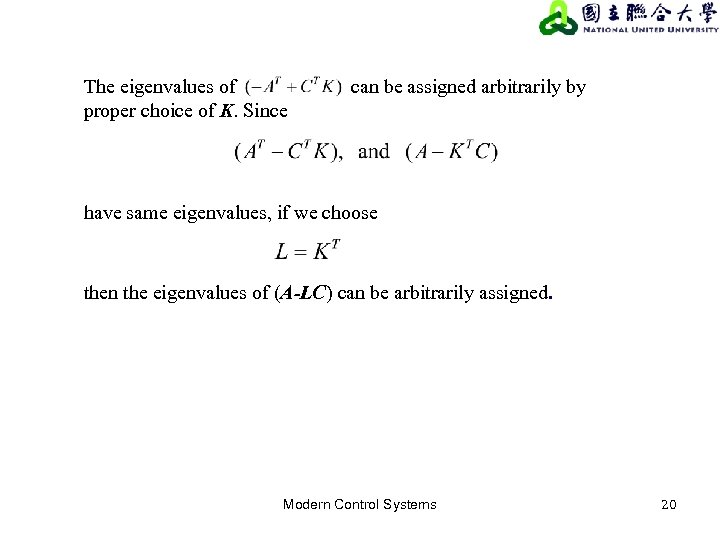The eigenvalues of proper choice of K. Since can be assigned arbitrarily by have same eigenvalues, if we choose then the eigenvalues of (A-LC) can be arbitrarily assigned. Modern Control Systems 20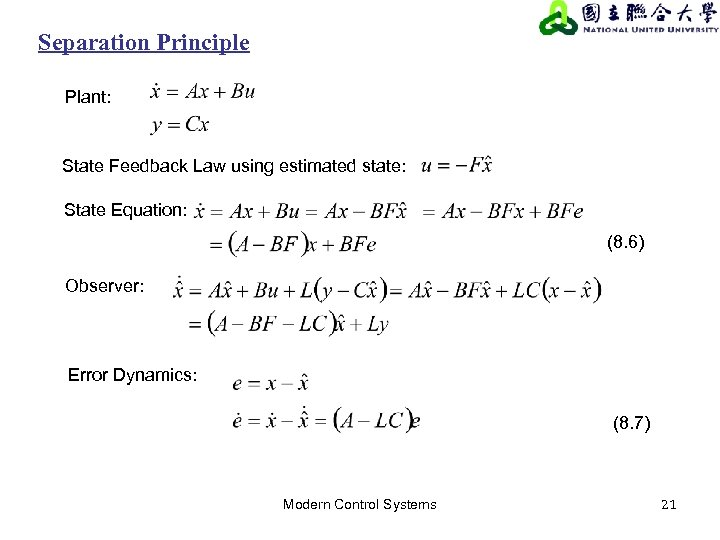Separation Principle Plant: State Feedback Law using estimated state: State Equation: (8. 6) Observer: Error Dynamics: (8. 7) Modern Control Systems 21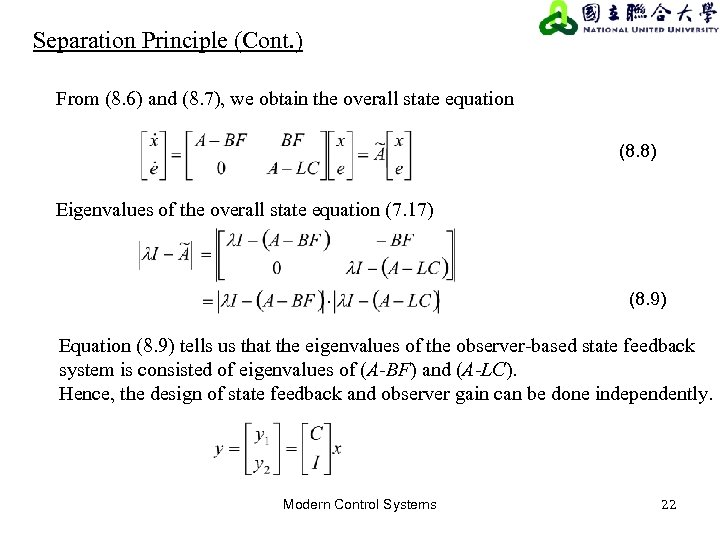Separation Principle (Cont. ) From (8. 6) and (8. 7), we obtain the overall state equation (8. 8) Eigenvalues of the overall state equation (7. 17) (8. 9) Equation (8. 9) tells us that the eigenvalues of the observer-based state feedback system is consisted of eigenvalues of (A-BF) and (A-LC). Hence, the design of state feedback and observer gain can be done independently. Modern Control Systems 22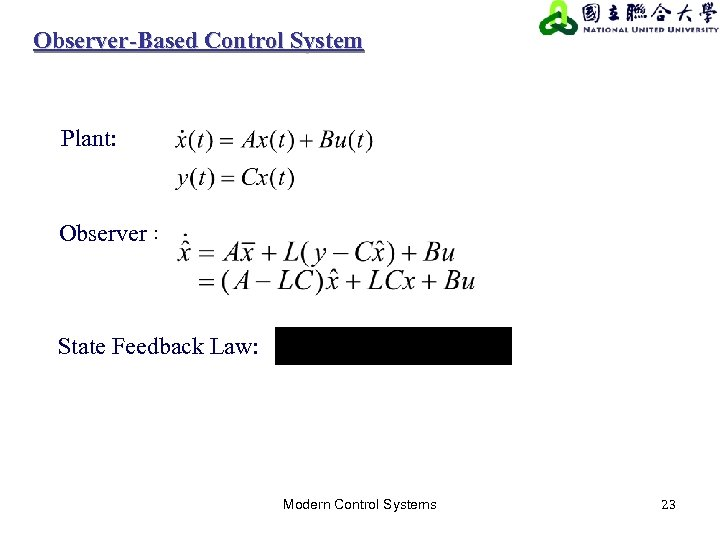Observer-Based Control System Plant: Observer： State Feedback Law: Modern Control Systems 23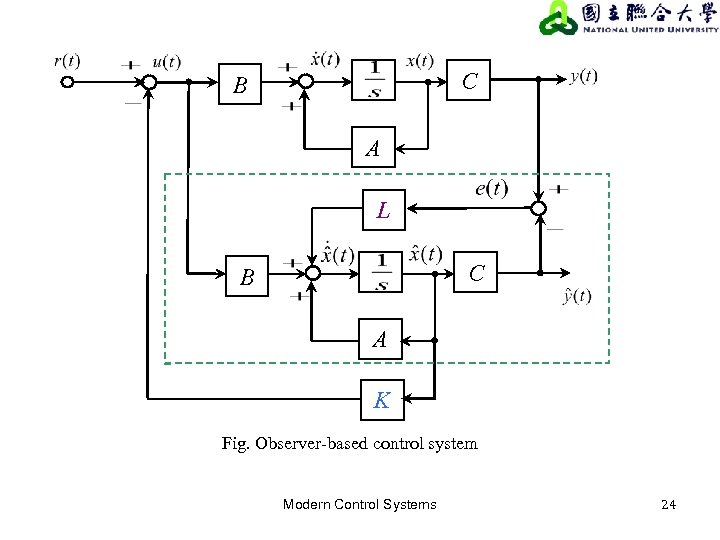C B A L C B A K Fig. Observer-based control system Modern Control Systems 24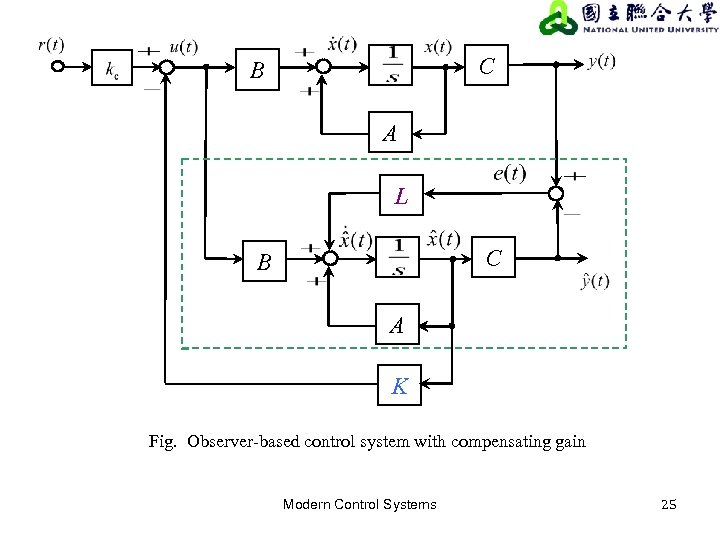C B A L C B A K Fig. Observer-based control system with compensating gain Modern Control Systems 25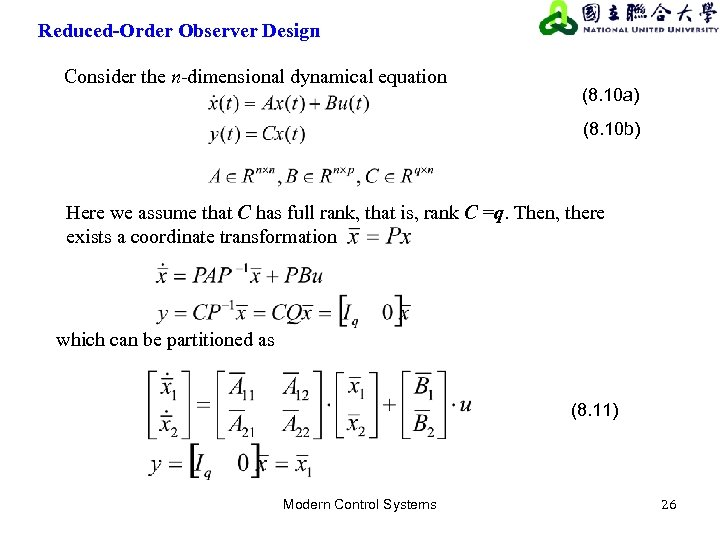Reduced-Order Observer Design Consider the n-dimensional dynamical equation (8. 10 a) (8. 10 b) Here we assume that C has full rank, that is, rank C =q. Then, there exists a coordinate transformation which can be partitioned as (8. 11) Modern Control Systems 26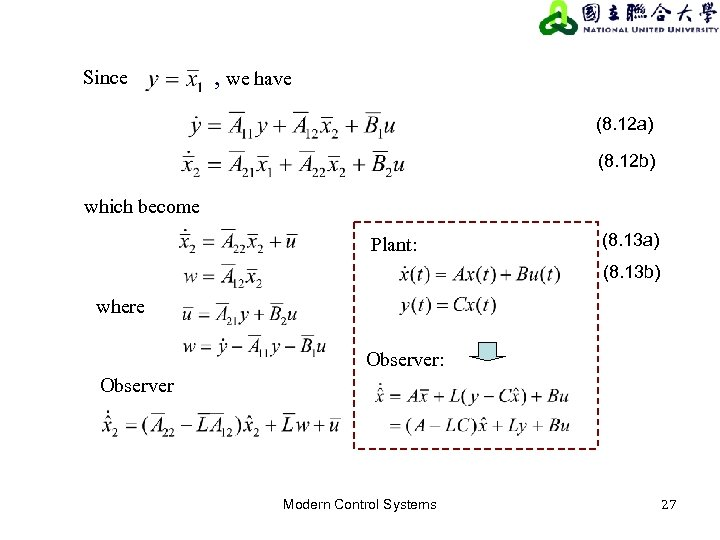Since , we have (8. 12 a) (8. 12 b) which become Plant: (8. 13 a) (8. 13 b) where Observer: Observer Modern Control Systems 27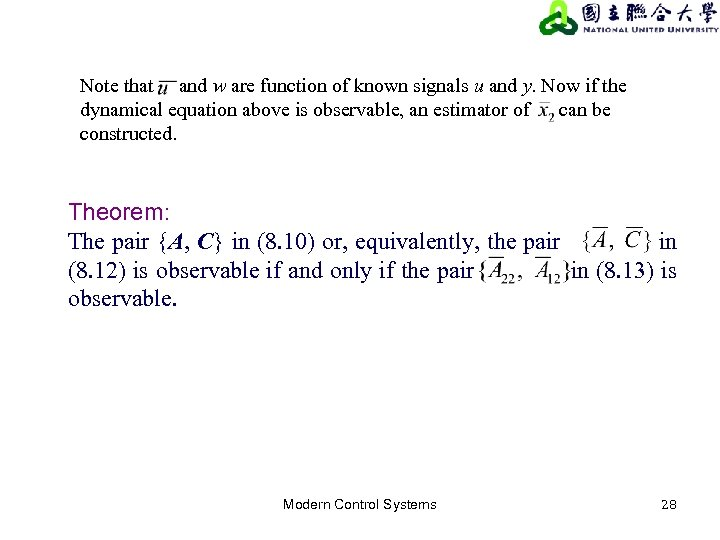Note that and w are function of known signals u and y. Now if the dynamical equation above is observable, an estimator of can be constructed. Theorem: The pair {A, C} in (8. 10) or, equivalently, the pair in (8. 12) is observable if and only if the pair in (8. 13) is observable. Modern Control Systems 28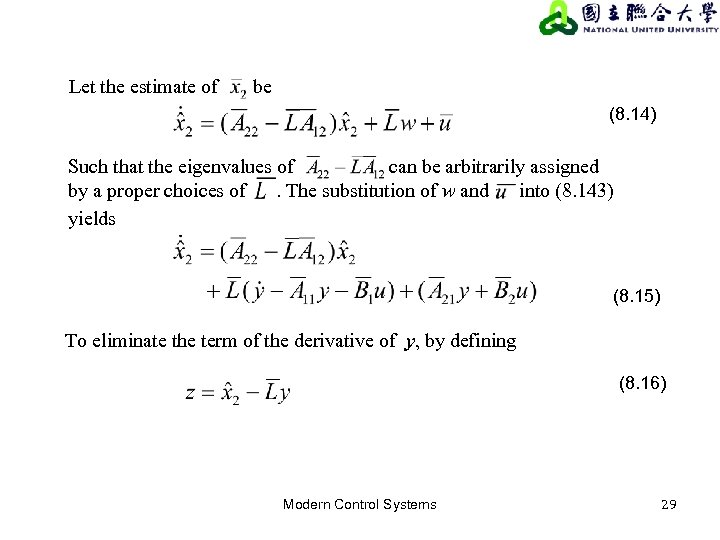Let the estimate of be (8. 14) Such that the eigenvalues of can be arbitrarily assigned by a proper choices of. The substitution of w and into (8. 143) yields (8. 15) To eliminate the term of the derivative of y, by defining (8. 16) Modern Control Systems 29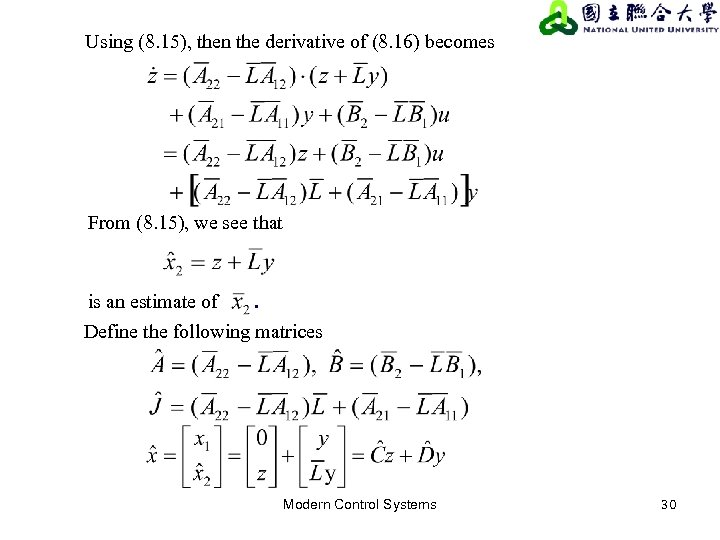Using (8. 15), then the derivative of (8. 16) becomes From (8. 15), we see that is an estimate of . Define the following matrices Modern Control Systems 30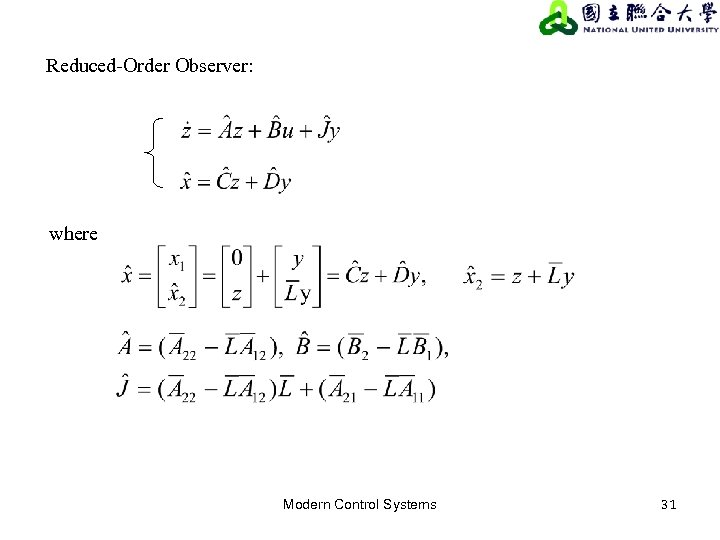Reduced-Order Observer: where Modern Control Systems 31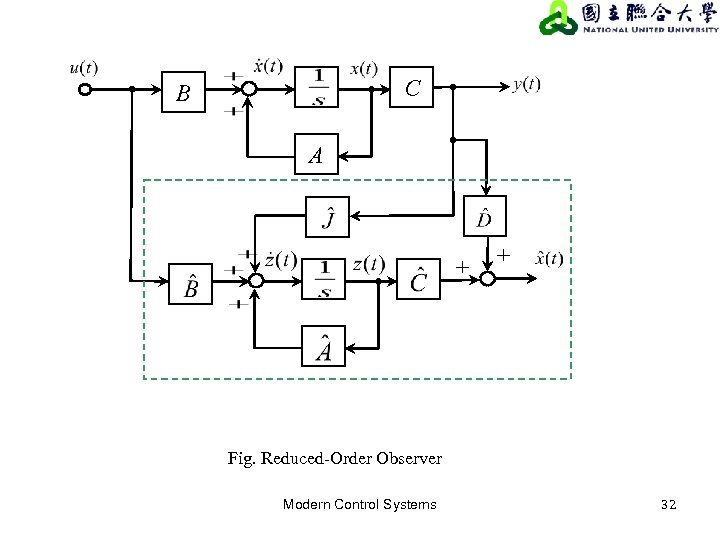C B A + + Fig. Reduced-Order Observer Modern Control Systems 32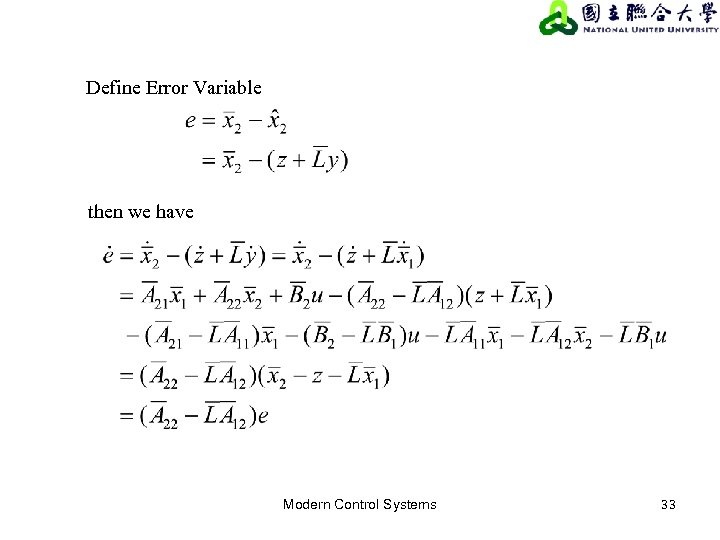Define Error Variable then we have Modern Control Systems 33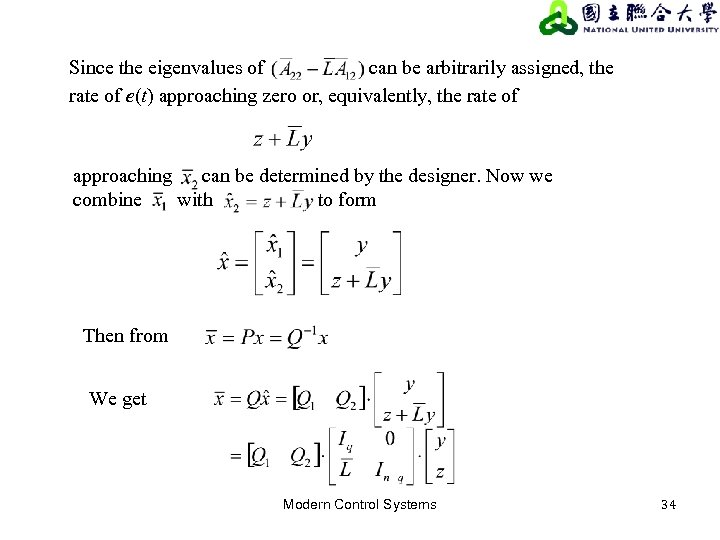Since the eigenvalues of can be arbitrarily assigned, the rate of e(t) approaching zero or, equivalently, the rate of approaching can be determined by the designer. Now we combine with to form Then from We get Modern Control Systems 34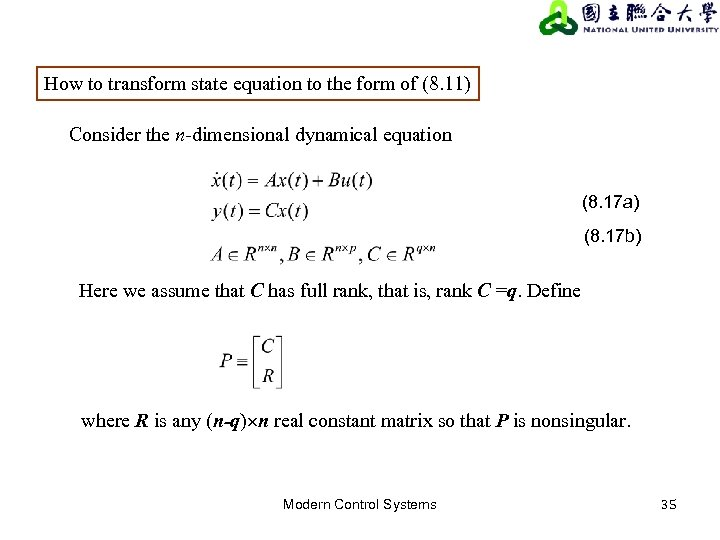How to transform state equation to the form of (8. 11) Consider the n-dimensional dynamical equation (8. 17 a) (8. 17 b) Here we assume that C has full rank, that is, rank C =q. Define where R is any (n-q) n real constant matrix so that P is nonsingular. Modern Control Systems 35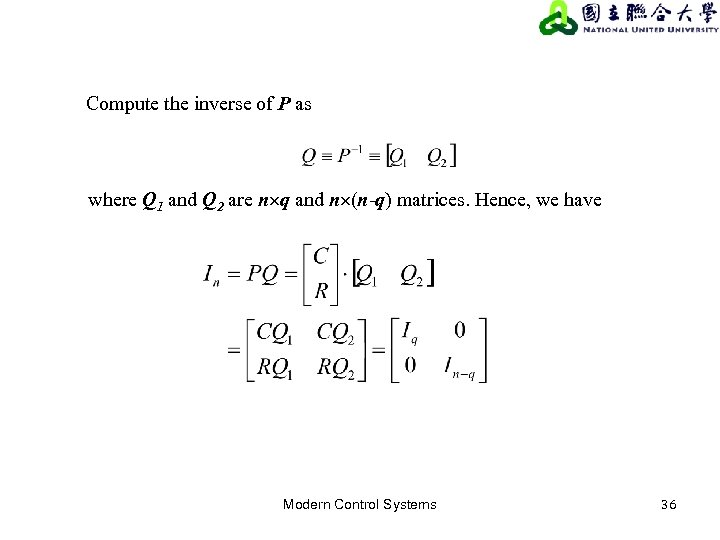Compute the inverse of P as where Q 1 and Q 2 are n q and n (n-q) matrices. Hence, we have Modern Control Systems 36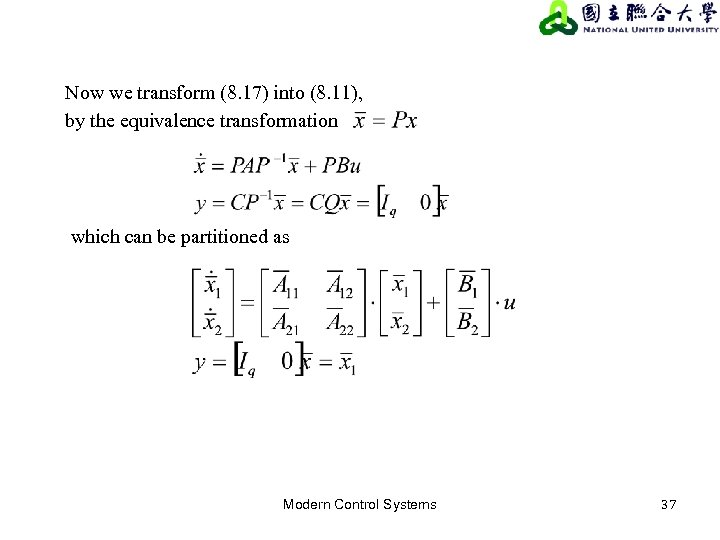Now we transform (8. 17) into (8. 11), by the equivalence transformation which can be partitioned as Modern Control Systems 37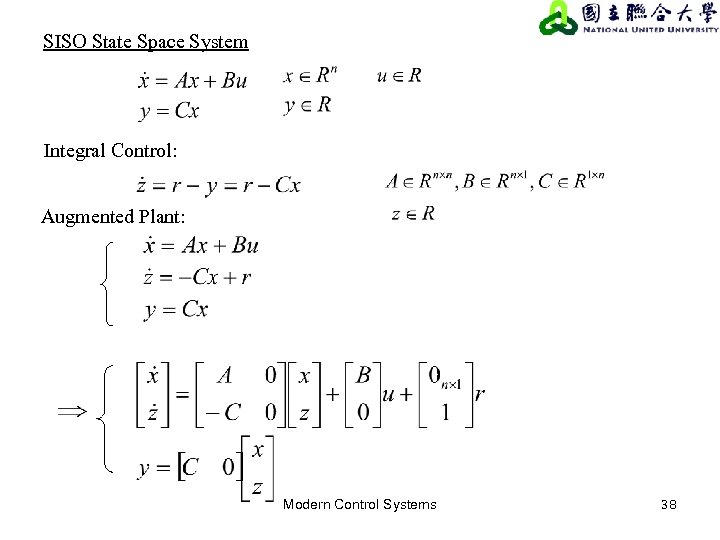SISO State Space System Integral Control: Augmented Plant: Modern Control Systems 38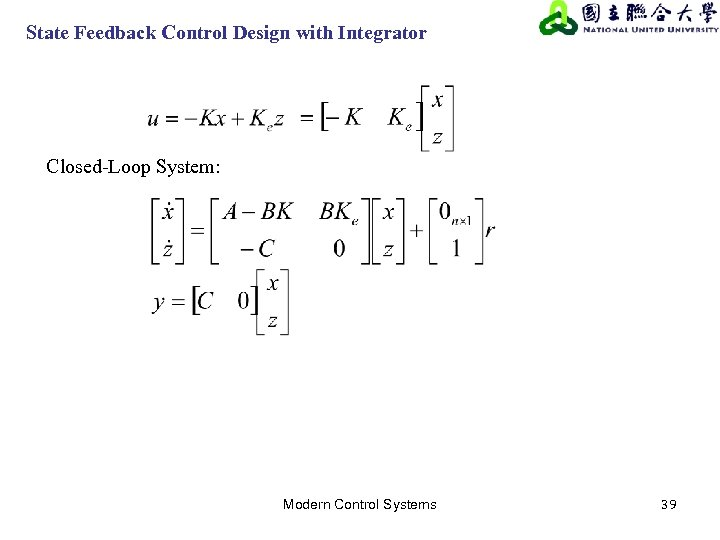State Feedback Control Design with Integrator Closed-Loop System: Modern Control Systems 39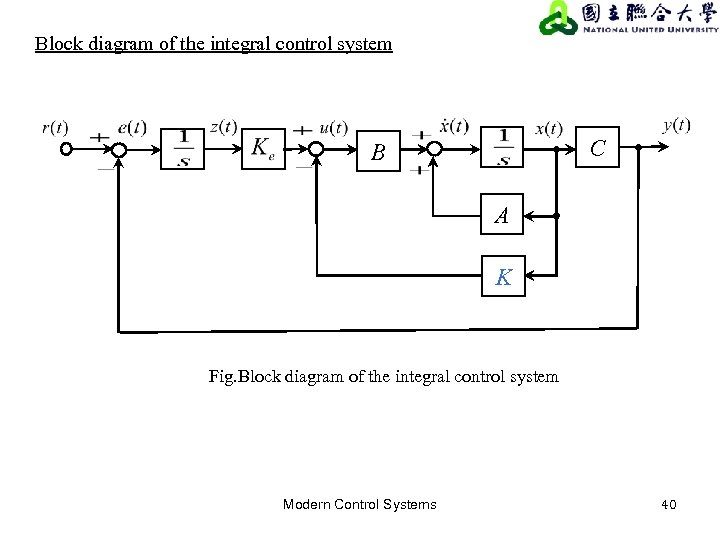Block diagram of the integral control system C B A K Fig. Block diagram of the integral control system Modern Control Systems 40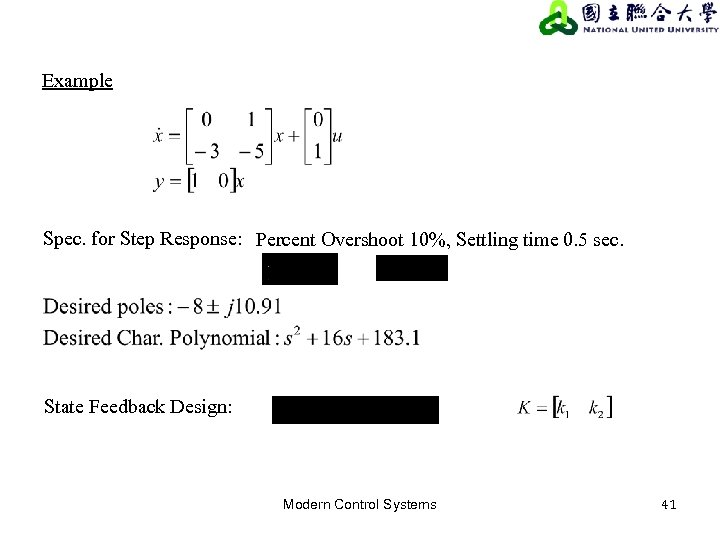Example Spec. for Step Response: Percent Overshoot 10%, Settling time 0. 5 sec. State Feedback Design: Modern Control Systems 41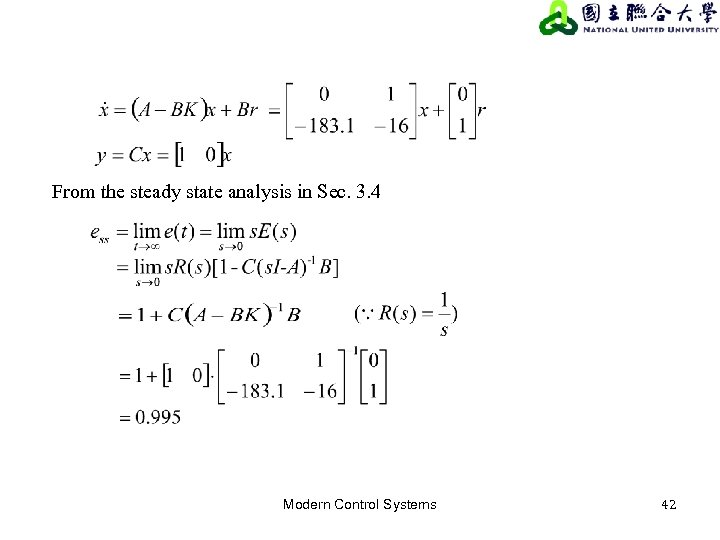From the steady state analysis in Sec. 3. 4 Modern Control Systems 42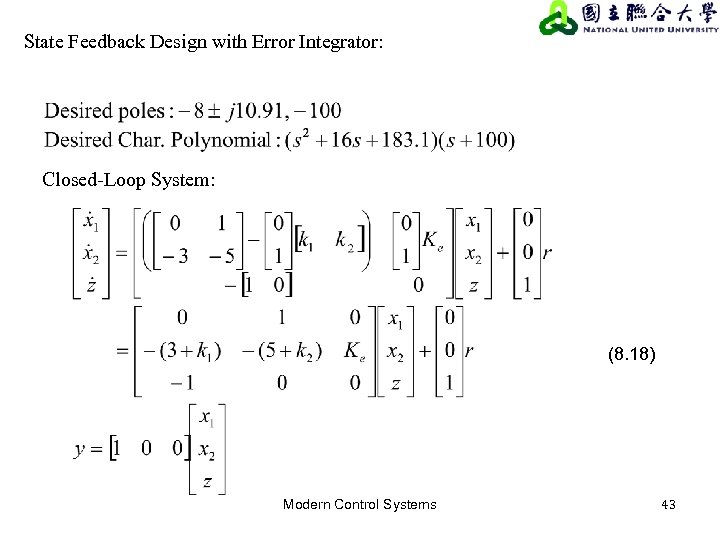State Feedback Design with Error Integrator: Closed-Loop System: (8. 18) Modern Control Systems 43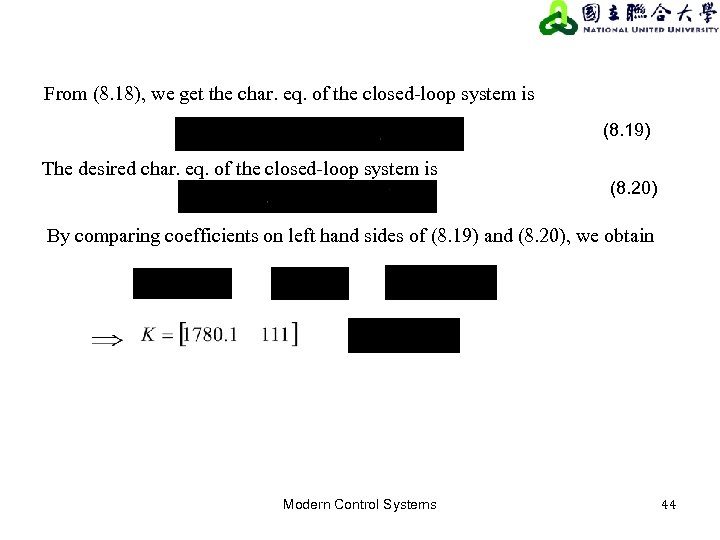From (8. 18), we get the char. eq. of the closed-loop system is (8. 19) The desired char. eq. of the closed-loop system is (8. 20) By comparing coefficients on left hand sides of (8. 19) and (8. 20), we obtain Modern Control Systems 44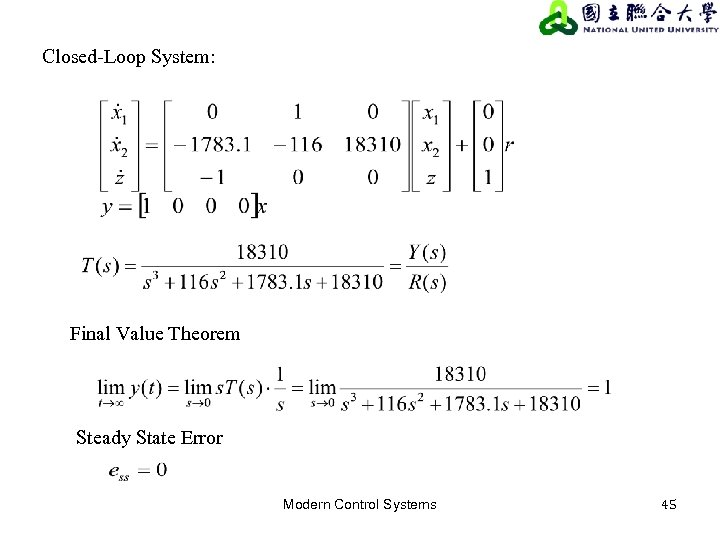Closed-Loop System: Final Value Theorem Steady State Error Modern Control Systems 45